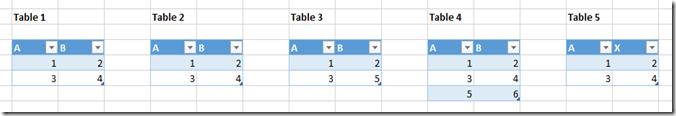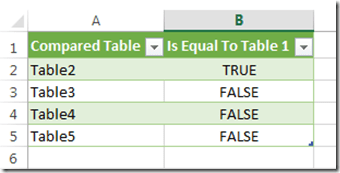# Comparing Tables In Power Query

An interesting Power Query nugget for you: you can compare two tables using the Value.Equals() function. For example, take the following worksheet with five Excel tables on it:The following Power Query query compares Table 1 with each of the other four tables and tells me whether they are identical or not:

`let`

`    Table1 = Excel.CurrentWorkbook(){[Name="Table1"]}[Content],`

`    Table2 = Excel.CurrentWorkbook(){[Name="Table2"]}[Content],`

`    Table3 = Excel.CurrentWorkbook(){[Name="Table3"]}[Content],`

`    Table4 = Excel.CurrentWorkbook(){[Name="Table4"]}[Content],`

`    Table5 = Excel.CurrentWorkbook(){[Name="Table5"]}[Content],`

`    Output = Table.FromRows(`

`                {`

`                {"Table2", Value.Equals(Table1, Table2)},`

`                {"Table3", Value.Equals(Table1, Table3)},`

`                {"Table4", Value.Equals(Table1, Table4)},`

`                {"Table5", Value.Equals(Table1, Table5)}`

`                },`

`                {"Compared Table", "Is Equal To Table 1"}`

`        )`

`in`

`    Output`

All the code here is doing is loading the five Excel tables and then outputting a single table that shows the result of Value.Equals() when you compare the first table with the other four. Here’s the output:I’ve tested this on tables sourced from SQL Server and quickly ran into a bug that crashed Power Query, but it seems as though Value.Equals() returns True when you pass it two identical tables and False when you pass it a table and a view which is just a SELECT * from that table. I wonder if there’s some extra metadata that allows Power Query to tell the difference between a table and a view? More research needed I think.

Overall this seems quite a handy trick to know about. This post has barely scratched the surface of what you can do with Value.Equals() though – you can compare any two values, not just tables, and you can specify your own function to do the comparison. As with so much of Power Query there’s a lot to learn… but that’s what makes it so fun!

### 8 responses

1.selfservicebi says:

Hat dies auf MS Excel | Power Pivot | DAX rebloggt.

•Patrick says:

Hi Chris,

in the example you build a table with the results “from scratch”. Is there the possibility to have a comparison with “joined” data in a merged query? Will say to have the comparison in a column at the end of a merged query to determine differences between columns. I didn’t manage to write a kind of if…then…else up to now…

Best regs
Patrick

•Chris Webb says:

You know, I have an idea how to do this but it needs testing. If my idea works I’ll write a blog post explaining it!

2.Jeff Nixon says:

Many thanks Chris!

Thanks to your post, I’m now using this technique when I optimize my M-code. As I rewrite, I compare the results from the already-validated original query output with that of the revised version:

let
Table1 = OriginalQueryName,
Table2 =RevisedQueryName
Output = Value.Equals(Table1, Table2)
in
Output

This ensures that I didn’t revise in such a way that the new output has some difficult-to-detect differences from the original.

This site uses Akismet to reduce spam. Learn how your comment data is processed.